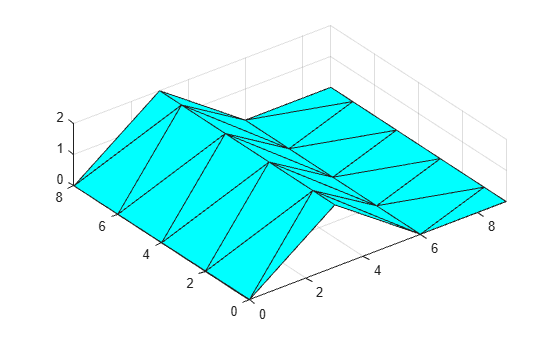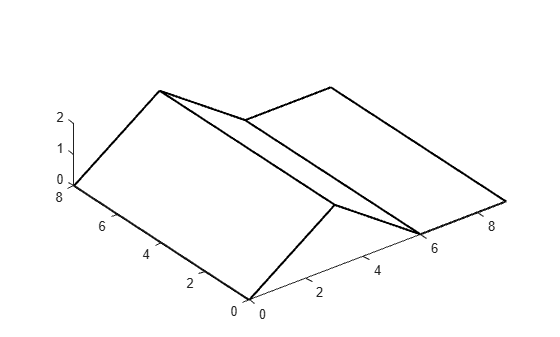featureEdges

Sharp edges of surface triangulation

Description

example

F = featureEdges(TR,theta) returns the feature edges in a 2-D triangulation according to an angle theta. This function is typically used to extract sharp edges in a surface mesh plot.

Examples

collapse all

Find and plot the feature edges of a surface.

Create a 2-D Delaunay triangulation.

x = [0 0 0 0 0 3 3 3 3 3 3 6 6 6 6 6 9 9 9 9 9 9]';
y = [0 2 4 6 8 0 1 3 5 7 8 0 2 4 6 8 0 1 3 5 7 8]';
DT = delaunayTriangulation(x,y);
T = DT.ConnectivityList;

Add elevations to the triangulation to create a surface and plot the surface.

z = [0 0 0 0 0 2 2 2 2 2 2 0 0 0 0 0 0 0 0 0 0 0]';
trisurf(T,x,y,z,'FaceColor','cyan')
axis equalCreate a new triangulation on the elevated surface, and compute and plot its feature edges using a filter angle of $\frac{\pi }{6}$.

TR = triangulation(T,x,y,z);
F = featureEdges(TR,pi/6)';
plot3(x(F),y(F),z(F),'k','LineWidth',1.5);
axis equalInput Arguments

collapse all

Triangulation representation for 2-D triangulations only, specified as a scalar triangulation or delaunayTriangulation object.

Data Types: triangulation | delaunayTriangulation

Filter angle in radians, specified as a scalar in the range [0,π]. featureEdges returns adjacent triangles that have a dihedral angle that deviates from π by an angle greater than theta.

Data Types: double

collapse all

Feature Edge

A feature edge is a triangulation edge that has any of the following attributes:

• The edge belongs to only one triangle.

• The edge is shared by more than two triangles.

• The edge is shared by a pair of triangles with angular deviation greater than the angle theta.

F is a two-column matrix whose rows correspond to a feature edge. The first element of each row is the identification number of the starting edge vertex, and the second element is the identification number of the ending edge vertex. A vertex identification is the row number of the corresponding vertex in the Points property.